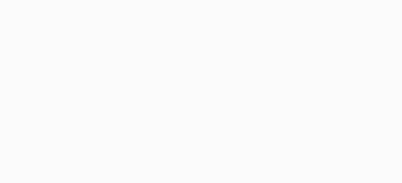#IBPS PO 2017 Practice Test – Quant Day 26

Hello Aspirants.

Welcome to Online Reasoning Section with the explanation in AffairsCloud.com. Here we are creating Best question samples for IBPS PO 2017. We have included Some questions that are repeatedly asked in bank exams !!!

IBPS PO 2017: Reasoning Test– 6 PM Every Day
IBPS PO 2017: Quants Test– 7 PM Every Day

Q(1-5) In the following questions two equations numbered I & II are given. You have to solve both equations and mark the option.

1. I. 50X² – 40X + 42 = 0
II. 10Y² + 41Y + 21 = 0
A. X > Y
B. X <Y
C. X ≥ Y
D. X ≤ Y
E. X = Y or relationship cannot be established
C. X ≥ Y
Solution:
X = 7/5, -6/10
Y = -3/5 , -7/2.

2. I. 2X² – 15√2X + 56 = 0
II. 2Y² – 17√2Y + 72= 0
A. X > Y
B. X <Y
C. X ≥ Y
D. X ≤ Y
E. X = Y or relationship cannot be established
D. X ≤ Y
Solution:
X = 7/√2, 8/√2
Y = 8/√2, 9/√2

3. I. √2X² + 8X – 64√2 = 0
II. √2Y² – 27Y + 81√2= 0
A. X > Y
B. X <Y
C. X ≥ Y
D. X ≤ Y
E. X = Y or relationship cannot be established
B. X <Y
Solution:
X = -8√2, 8/√2
Y = 9/√2, 9√2

4. I. X² + 25X + 156 = 0
II. Y² + 29Y + 210 = 0
A. X > Y
B. X <Y
C. X ≥ Y
D. X ≤ Y
E. X = Y or relationship cannot be established
X > Y
Solution:
X= -12,- 13
Y = -14,- 15

5. I. 3X + 4Y = 64
II. 2X + 3Y = 56
A. X > Y
B. X <Y
C. X ≥ Y
D. X ≤ Y
E. X = Y or relationship cannot be established
B. X <Y
Solution:
X = -32, Y = 40

Q(6-10) Study the given table and answer the following questions1. If the difference between the Profits made by Shop A and Shop B on Mouse is equal to the Profit earned by Shop C on Calculator then his Profit percent is?
A. 50/13%
B. 49/11%
C. 50/11%
D. 50/23%
E. Cannot be determined
C. 50/11%
Solution:
2500*(13-9)/100 = Rs 100
2300 = 2200(100+x)/100
X = 50/11%

2. What is the average profit made Shop A, B and C on Mouse?
A. 345
B. 335
C. 325
D. 315
E. None
C. 325
Solution:
25*13 = 325

3. Shop A has made Maximum Profit on which article?
A. Mouse
B. Keyboard
C. Hard Disk
D. Calculator
A. Mouse
Solution:
Checking by observation
Mouse : 25*13 = 325
Hard Disk: 26*12 = 312

4. If the total profit made by Shop A, B and C on Hard Disk is Rs.988 then what is the profit of B on Hard Disk?
A. 276
B. 286
C. 296
D. 216
E. None
B. 286
Solution:
26*(12+x+15) = 988
X = 11%
Profit = 26*11 = 286

5. What is the difference between total profits made Shop A, B and C on Head Phones and Keyboard?
A. 44
B. 54
C. 64
D. 66
E. None
B. 54
Solution:
23*(11+18+13) – 24*(12+18+8)
966 – 912 = 54

AffairsCloud Recommends Oliveboard Mock Test

AffairsCloud Ebook - Support Us to Grow